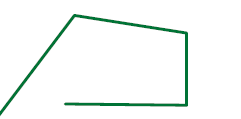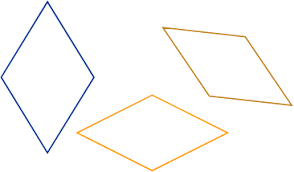Polygons & More
Polygons Only
100What is NOT a polygon?

100

This is the name for any 4 sided polygon.

100

This quadrilateral has 4 right angles and all 4 sides are the same length.

What is a square?

200

This angle is greater than 90*.

What is an obtuse angle?

200What is a polygon?

200

This is any quadrilateral that has 2 pairs of parallel lines.

What is a parallelogram?

300

This angle is less than 90*.

What is an acute angle?

300

Shapes are flat and closed.

No circles.

Can have different numbers of sides.

All sides are straight.

What is a polygon?

300

A 4-sided flat shape with straight sides where all interior angles are right angles (90°).

What is a rectangle?

400

This is the angle at the edge of the whiteboard.

What is a right angle?

400

A 4-sided flat shape with straight sides where all interior angles are right angles (90°).

What is a rectangle?

400

A parallelogram with opposite equal acute angles, opposite equal obtuse angles, and four equal sides.What is a rhombus?

500

It has sides, but it is open.

What is NOT a polygon?

500

A closed two-dimensional (flat) shape with three or more sides.

What is a polygon?

500

A quadrilateral that has only one pair of parallel lines.

What is a trapezoid?

Click to zoom